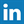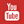# FreBlogg

## Factorial of a number in C & C++ | The Simplest Way

13:19 Posted by DurgaSwaroop

Factorial of a number is a very commonly used function and so, not surprisingly programmers run in to this quite often. But, there is no direct function we can call to do this for us. So, each time i have to use this, i end up writing a 5-10 line code using recursion or some other method and then call that for the factorial, which is pretty boring.

But, I have found a very easy way to implement factorial with a built-in function of "math.h" called tgamma(). So, lets see how to implement this program
```#include <math.h>
#include <stdio.h>

double fact(double x)
{
return tgamma(x+1);
}
int main()
{
printf("%f %f\n", fact(3.0), fact(5.0));
return 0;
} ```
So, that is a very easy implementation of Factorial function. This factorial function return double values as you can see in the following output.

And, one more thing you can notice, if you are using gcc compiler which is available by default in all the Linux and Unix based systems, you might want to use the -lm as shown in the above image. Otherwise you'll get an error as shown below.

In  other compilers i tried, i didn't see this. So, if you are using gcc take a note of that. You have to use that when ever you use a function from "math.h"

Most of the times we want to work with int instead of double. So, to get int outputs, you just have to typecast the double value to int and then you'll get the output in int.

So, That is all for this article. If you like this article you might like our other articles too about Programming. So, check them out. Also stay tuned for a lot of other interesting articles.

As, always, Have a happy reading and Stay Awesome !

-------------------------------------------------------------------------------------------------
Follow our blog posts @ Follow. So that you won't miss any interesting post and also to be the first to know the answers to many interesting questions.
Head over to my You Tube channel for some interesting tutorials @ You Tube1.